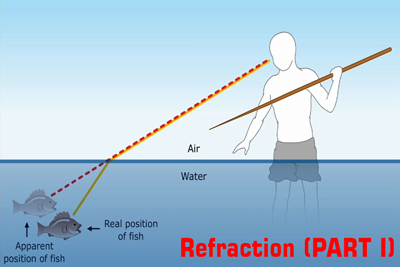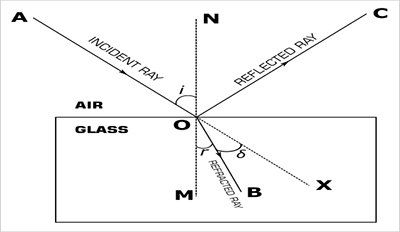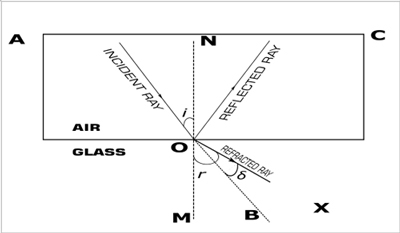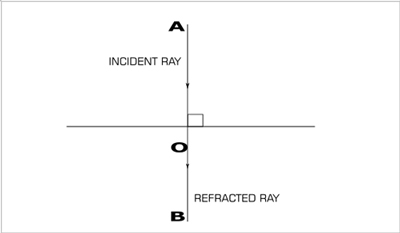Refraction of light - Physics
18-01-2020    623 timesWhen a light ray passes through two different homogeneous and transparent medium, then the direction of the path of the light change on the surface of the second medium. This phenomenon is a known as refraction of light.In figure AO represents the incident ray, OC is the reflected ray and OB is the refracted ray. NOM is the normal at the point of the incident O. ∠AON is the angle of the incident i and ∠BOM is the angle of refraction r. The actual path of the incident ray will be AOX if the medium remains the same. Here the angle between the actual path of the incident ray and refracted ray is known as deviation , denoted by δ. Therefore, δ =i – r.

By experimentally observation, we found three different situations when light passes from one medium to another.1. When a ray of light refracts and meets the following conditions-
1. Travels from a rarer medium to a denser medium (Say, from to air to glass)
2. It bends towards the normal

Then the angle of the incident i greater than the angle of refraction r. i.e. deviation(δ) = i – r.

2. When a ray of light refracts and meets the following conditions-1. Travels from a denser medium to a rarer medium (Say, from to glass to air).
2. It bends towards the normal.

Then the angle of refraction r is greater than the angle of the incidenti. i.e deviation(δ) = r – i.

3. When a ray of light refracts and meets the following conditions
1. The angle of incidence ∠i = 0° that is, such ray suffers no bending at the surface.

Then, the deviation is to equals.### Cause of refraction

When a ray of light passes from one medium to another medium, then its direction (or path) changes because of the change in the speed of light from one medium to another. For normal incidence (∠i=0°) although direction does not change but the speed of light certainly changes.